Note: The other languages of the website are Google-translated. Back to English

## Excel IF 功能

IF函数是Excel工作簿中最简单，最有用的函数之一。 它根据比较结果执行简单的逻辑测试，如果结果为TRUE，则返回一个值，如果结果为FALSE，则返回另一个值。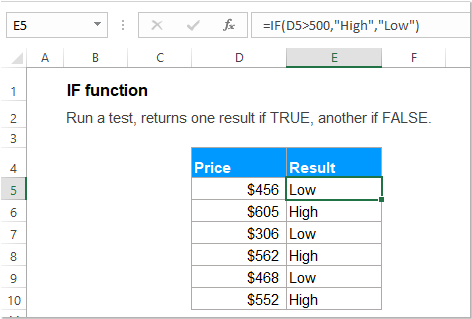#### 语法：

Excel中IF函数的语法为：

=IF (logical_test, [value_if_true], [value_if_false])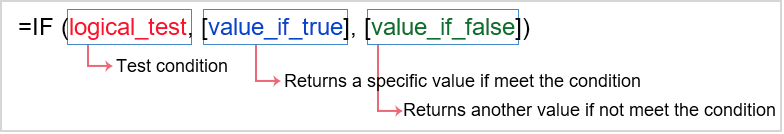#### 参数：

• logical_test：必填。 这是您要测试的条件。
• value_if_true： 可选的。 如果逻辑测试结果为TRUE，则要返回的特定值。
• value_if_false： 可选的。 如果逻辑测试结果为FALSE，则要返回的值。

1。 如果 value_if_true 省略：

• 如果 value_if_true IF函数中省略了参数，例如，仅当logic_test之后是逗号时，满足条件时它将返回零。 例如： =IF(C2>100,, "Low ").
• 如果要满足条件，则要使用空白单元格而不是零，则应在第二个参数中输入双引号“”，如下所示： =IF(C2>100, "", "Low").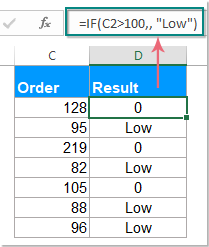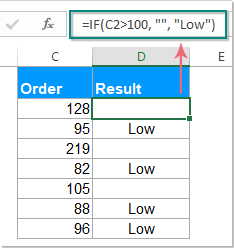2。 如果 value_if_false 省略：

• 如果 value_if_false IF函数中省略了参数，当不满足指定条件时，它将返回FALSE。 如： =IF(C2>100, "High").
• 如果在value_if_true参数后面放置逗号，则当不满足指定条件时它将返回零。 如： =IF(C2>100, "High" ,).
• 如果在第三个参数中输入双引号“”，则不满足条件将返回一个空单元格。 如： =IF(C2>100, "High" , "").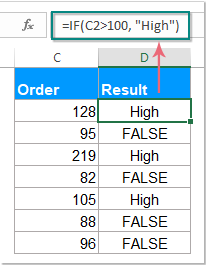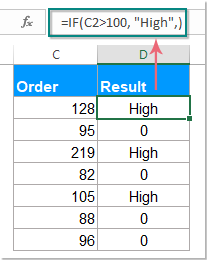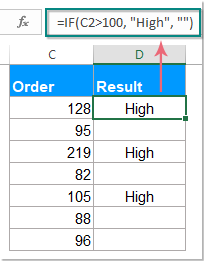#### 例子：

##### 示例1：对数字使用简单的IF函数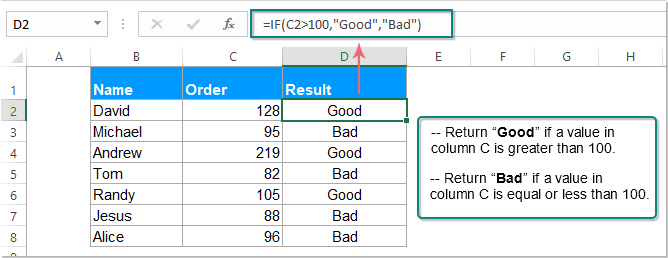##### 示例2：将IF函数用于文本值

=IF(C2="completed", "No", "Yes")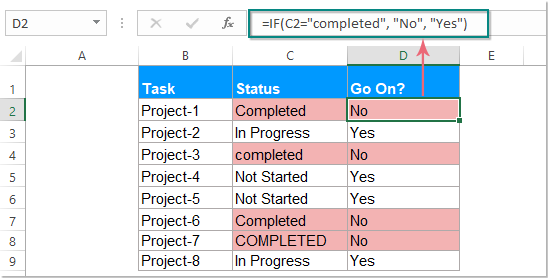=IF(EXACT(C2,"COMPLETED"), "No", "Yes")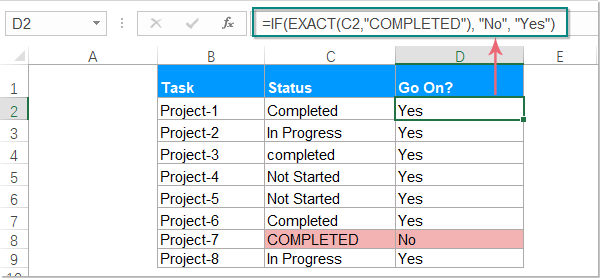=IF(ISNUMBER(SEARCH("comp",C2)), "No", "Yes")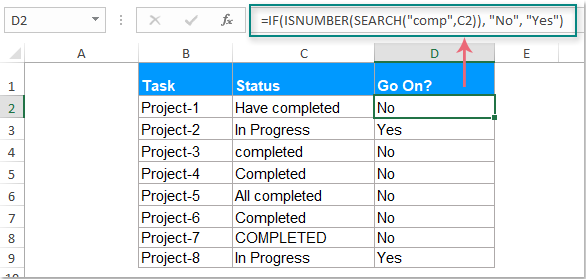• 1. SEARCH函数适用于不区分大小写的文本，如果要检查区分大小写的文本，则应将SEARCH函数替换为FIND函数。 像这样：=IF(ISNUMBER(FIND("comp",C2)), "No", "Yes")
• 2.文本值作为IF公式中的参数，必须将其括在“双引号”中。

##### 示例3：将IF函数用于日期值

=IF(D4>DATEVALUE("4/15/2019"), "Yes", "No")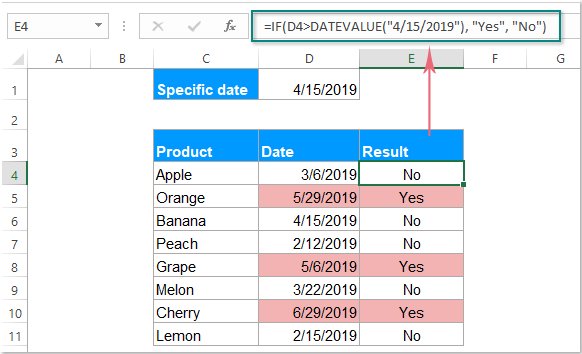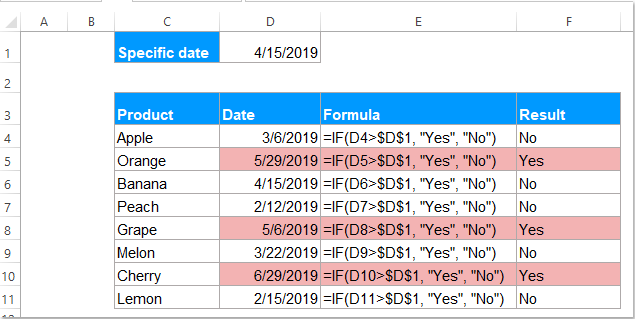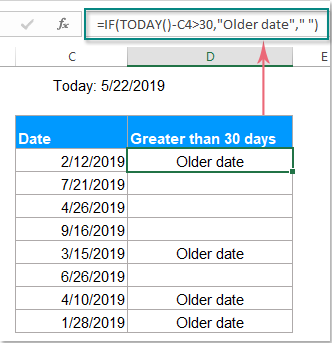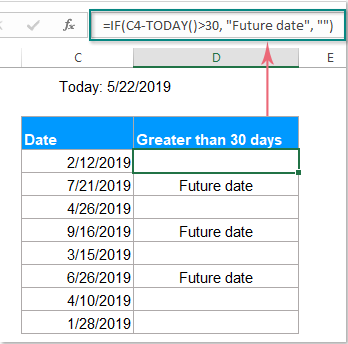=IF(C4-TODAY()>30, "Future date", IF(TODAY()-C4>30, "Older date", ""))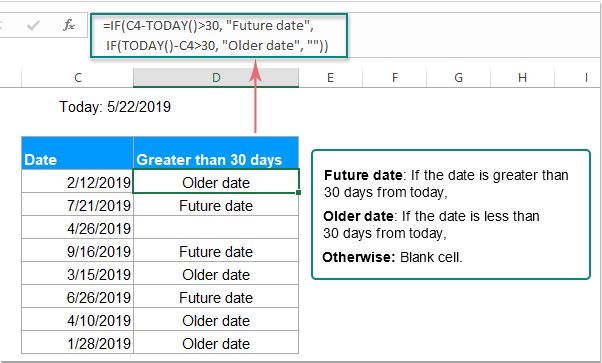##### 示例4：将IF函数与AND，OR函数一起使用

=IF(AND(B4="Red",C4="Small", D4>200),"Yes","No")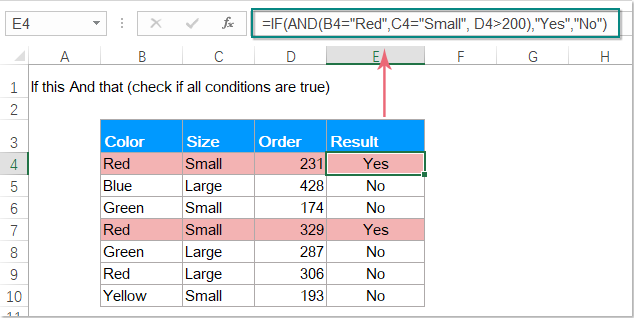=IF(OR(B4="Red",B4="Blue"),"Yes","No")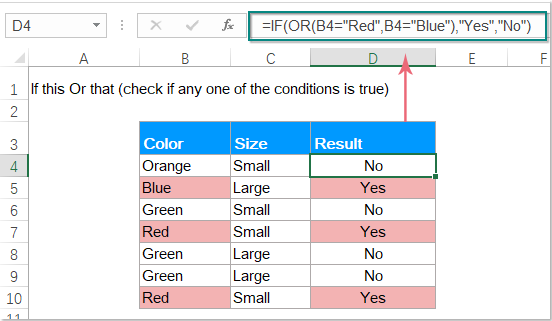• Condition 1: Column B = “Red” and Column D > 300;
• Condition 2: Column B = “Blue” and Column D > 300.

=IF(AND(OR(B4="Red",B4= "Blue"), D4>300), "Match", "No")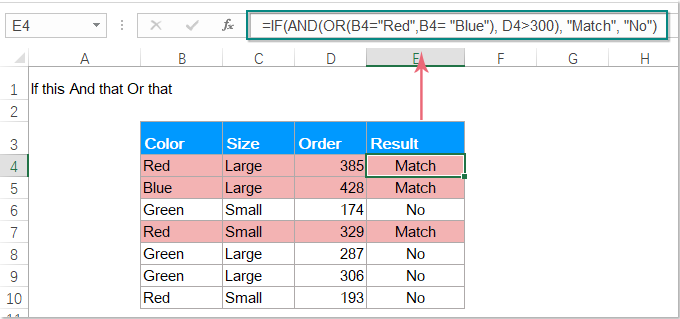##### 示例5：使用嵌套IF函数

IF函数用于测试条件，如果满足条件则返回一个值，如果不满足则返回另一个值。 但是，有时候，您需要同时检查多个条件并返回不同的值，您可以使用Nested IF解决此工作。

Excel中的嵌套IF函数的语法为：

=IF (condition1, result1, IF (condition2, result2, IF (condition3, result3,…)))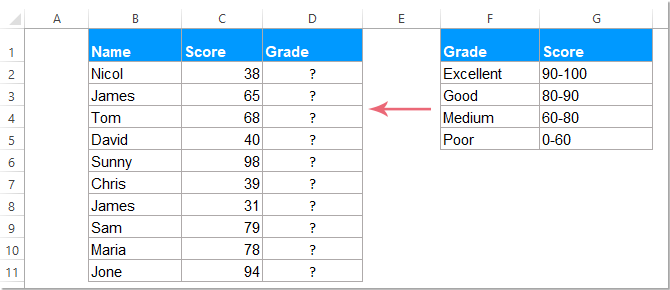=IF(C2>=90, "Excellent", IF(C2>=80, "Good", IF(C2>=60, "Medium", "Poor")))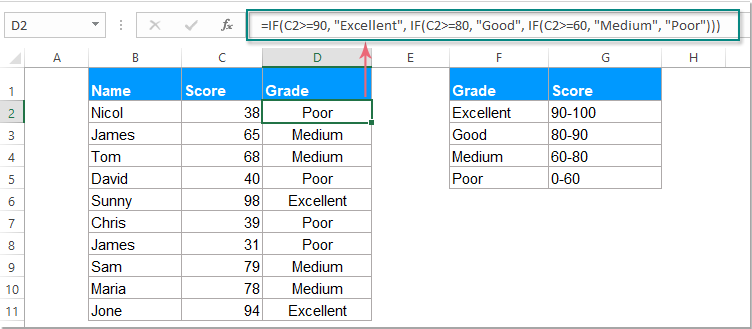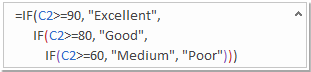IF(check if C2>=90, if true - returns "Excellent", or else IF(check if C2>=80, if true - returns "Good", or else IF(check if C2>=60, if true - returns "Medium", if false - returns "Poor")))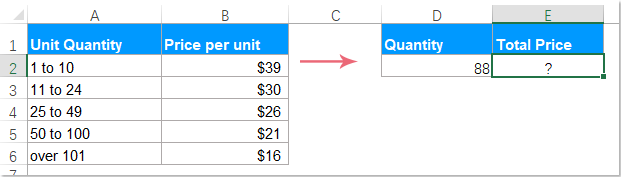=D2*IF(D2>=101,16, IF(D2>=50, 21, IF(D2>=25, 26, IF( D2>=11, 30, IF(D2>=1, 39, "")))))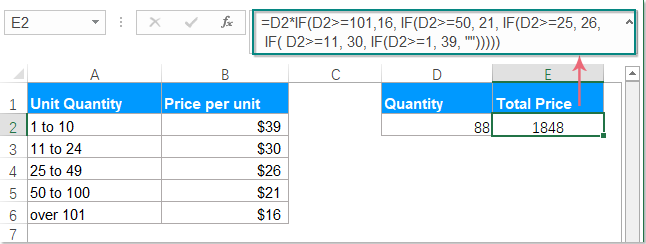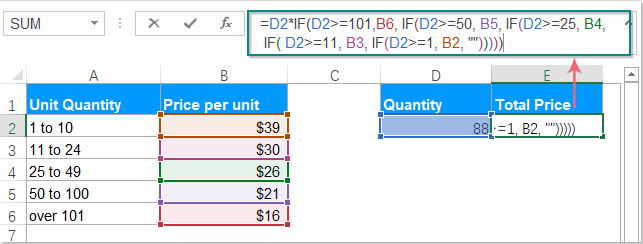操作者 意 使用案列 描述 > 比...更棒 =IF(A1>10, "OK",) 如果单元格A1中的数字大于10，则该公式返回“ OK”；否则，该公式将返回“ OK”。 否则返回0。 < 小于 =IF(A1<10, "OK", "") 如果单元格A1中的数字小于10，则公式返回“ OK”；否则，该公式将返回“ OK”。 否则，返回一个空单元格。 >= 大于或等于 =IF(A1>=10, "OK", "Bad") 如果单元格A1中的数字大于或等于10，它将返回“ OK”；否则，将返回“ OK”。 否则，显示“错误”。 <= 小于或等于 =IF(A1<=10, "OK", "No") 如果单元格A1中的数字小于或等于10，则返回“ OK”；否则，返回“ XNUMX”。 否则，返回“否”。 = 等于 =IF(A1=10, "OK", "No") 如果单元格A1中的数字等于10，则返回“ OK”；否则，返回“ XNUMX”。 否则显示“否”。 <> 不等于 =IF(A1<>10, "No", "OK") 如果单元格A1中的数字不等于10，则公式返回“否”； 否则-“确定”。

### 最佳办公效率工具

#### Kutools for Excel-帮助您从人群中脱颖而出

• 专为1500个工作方案而设计，可帮助您解决80％的Excel问题。
• 每天减少成千上万的键盘和鼠标点击，减轻您疲倦的眼睛和手部的疲劳。
• 在3分钟内成为一名Excel专家。 不再需要记住任何痛苦的公式和VBA代码。
• 30天无限制免费试用。 60天退款保证。 免费升级和支持2年。#### Office选项卡-在Microsoft Office（包括Excel）中启用选项卡式阅读和编辑

• 一秒钟即可在数十个打开的文档之间切换！
• 每天为您减少数百次鼠标单击，告别鼠标手。
• 查看和编辑多个文档时，可将您的工作效率提高50％。
• 像Chrome，Firefox和新的Internet Explorer一样，为Office（包括Excel）带来高效选项卡。0  产品特性